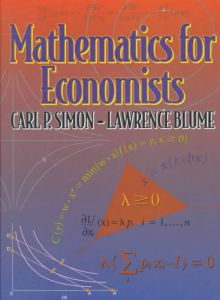## Monday, September 10, 2012

### Mathematical Foundations for Finance PDF SLIDESMathematical Foundations for Finance

Instructor: Phil Dybvig
Textbook: Mathematics for Economists by Carl Simon and Lawrence Blume.
Download Slides from here

Probability and Statistics, part 1 (2 lectures)
...pdf slides size 96K
...Problem set 1 size 21K, due Tue Sept 13

Calculus Review and Probability and Statistics, part 2 (2 lectures)
...calculus review pdf slides size 56K
...probability and statistics, part 2 pdf slides size 42K

Vectors and Matrices (2 lectures)
...matrix algebra ppt slides size 1184K
...eigenvalues and eigenvectors pdf slides size 50K

Multivariable Calculus and Optimization (4 lectures)
...multivariate calculus ppt slides size 756K and pdf slides size 28K (download both)
...optimization ppt slides size 1024K
...Kuhn-Tucker notes pdf notes 29K